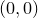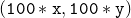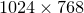## A.3.2 Writing a short example talk

The infrastructure is now completely set up, and you are ready to start designing slides. We will now design an example talk with three slides.

1. Run the script compile and leave it running in the background. Pyxplot will then re-run the scripts describing your slides whenever you edit them.

2. As an example, we will now make a title slide. Paste the following script into the file scripts/0001:

set output 'slides/0001.gif'

text '\parbox[t]{10cm}{\center \LARGE \bf \
A Tutorial in the use of Pyxplot \\ \
to present Talks \
} ' at x*50, y*75
text '\Large \bf Prof A.N.\ Other' at x*50, y*45
text '\parbox[t]{9cm}{\center \
Director, \\ \
Atlantis Island University \
} ' at x*50, y*38
text 'Annual Lecture, 1st January 2010' at x*50, y*22



Note that the variables x and y are defined to be 1 per cent of the width and height of your slides respectively, such that the bottom-left of each slide is atand the top-right of each slide is at.

3. Next we will make a second slide with a series of bullet points. Paste the following script into the file scripts/0002:

set output 'slides/0002.gif'

text '\Large \textbf{Talk Overview}' at x*50, y*92
text "\parbox[t]{9cm}{\begin{itemize} \
\item Setting up the Infrastructure. \
\item Writing a Short Example Talk. \
\item Conclusion. \
\end{itemize} \
} " at x*50 , y*60

set textcol cyan
text '{\bf With thanks to my collaborator, \
Prof Y.E.\ Tanother.}' at x*50,y*15


4. Finally, we will make a third slide with a graph on it. Paste the following script into the file scripts/0003:

set output 'slides/0003.gif'

text '\Large \bf The Results of Our Model' at x*50, y*92
set axescolour yellow ; set nogrid
set origin x*17.5, y*20 ; set width x*70
set xrange [0.01:0.7]
set xlabel '$x$'
set yrange [0.01:0.7]
set ylabel '$f(x)$'
set palette Red, Green, Orange, Purple

set key top left
plot x t 'Model 1', exp(x)-1 t 'Model 2', \
log(x+1) t 'Model 3', sin(x) t 'Model 4'


5. To view your slides, run the script make_slides. Afterwards, you will find your slides as a series ofpixel jpeg images in the directory slides_cropped. If you have the Quick Image Viewer (qiv) installed, then you can view them as follows:
qiv slides_cropped/*